Home | Previous | Next Lesson

This Lesson Can Be Printed See Instructions Below

# Moving Averages That Work

Of all the things that are written about in trading probably the most common is the Moving Average. It is the starting point for most tutorials and the hope of every new trader that he will find a moving average that he can apply to a chart and that will make money.

 I don't know what it is about the moving average that fascinates traders, we have all been enticed by their simplicity. I am sure at some point you have either tried a moving average crossover system or a close above or below the moving average as a signal. The problem as I am sure you have come to realize is the whipsaw that results when you try and follow the signals mechanically. That is to say you take every signal that is presented. In this lesson I shall introduce you to what I believe is a much more reliable moving average. First lets just make sure you know what a moving average is.

What Is A Moving Average

A moving average is simply the average number of a given sequence of numbers. If you have 5 numbers in a sequence e.g. 4,8,6,4,7 the average is 5.8; you get this by first adding all the numbers in the sequence and then dividing that number by the amount of numbers in the sequence.
4+8+6+4+7=29
29/5=5.8

Let's say you were tracking the daily close of a particular security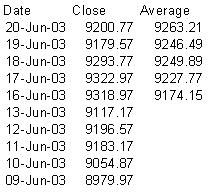The table above is a 5 period simple moving average. The average of 9263.21 is the average of the previous 5 closes.

If you were to see this on a chart it would look like this.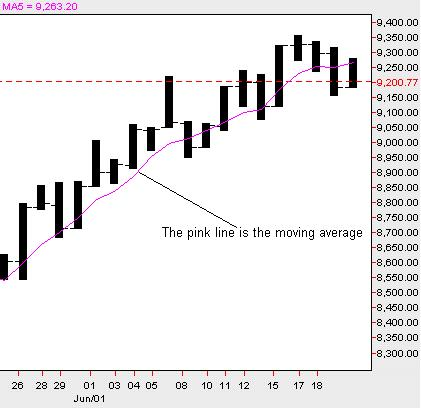Here's the problem with a lot of moving averages. The average you use may not be representative of the market as it actually is.

Let's say you have 5 cars in a parking lot. 4 of the cars are worth \$10,000 and the other one is worth \$50,000. The average of all the cars is \$18,000 right. But is that a fair representation of all the cars in the lot?

Most of the cars are \$10,000 but because one car is aberrantly priced it effects the whole average. The same is true of the markets. You can sometime have a huge move in the market that effects the moving average and because you may for example be using a 5 period moving average it will effect the average for the next 5 days even though the next 4 days may have a very small range. You won't see an accurate refection of the market until the sixth day when the large range day drops off.

To overcome this there have been many attempts at varying the way the moving average is constructed.

Types Of Averages

Simple Moving Average
A simple, or as it is sometimes called an arithmetic, moving average is calculated by adding any given number sequence and dividing that number sequence by the total numbers in that sequence.

Weighted Moving Average
The weighted moving average places more weight on recent data and less weight on past data. The weighted moving average is calculated by placing more emphasis (weight) on each of the preceding days data with the most weight on the most recent data. With a 5-day moving average there will typically be 5 times more weight on today's data than 5 days previously.

Exponential Moving Average

The exponential moving average is similar to the weighted moving average inasmuch as it places more emphasis on recent data. Typically a % of the most recent data is calculated and deducted from the previous data in the sequence.

There are many other types of moving averages but the above are the most common. It is interesting to note that in all the studies I have read there is no significant difference in the type of moving average used over time. The longer the look back period the less difference there is in the moving average.

The main problem I have found with new traders is that they will use a moving average for entry signals regardless of market conditions. Moving averages are best used for trending markets. Using moving averages in a market that is in consolidation will most likely lead to loss.

Enter The Displaced Moving Average

First let me tell you that displaced moving averages are not the Holy Grail. They have however helped me a lot in my trading.
What Is A Displaced Moving Average
A displaced moving average is simply a simple moving averaged moved forward in time.

The great advantage of this is that you will know what average you are using in advance. If you are using a 3 period moving average displaced once then you will know the moving average you will be using tomorrow before tomorrow comes.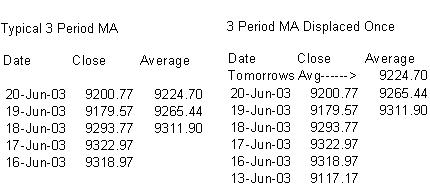In the chart below there are two moving averages. The black line is a typical 20 period moving average. The green line is a 20 period moving average displaced 5 times into the future.

You can see that in this up trend there are fewer closes below the green line thus keeping you in the trade longer. With less whipsaw you don't get kicked out of the trade as often.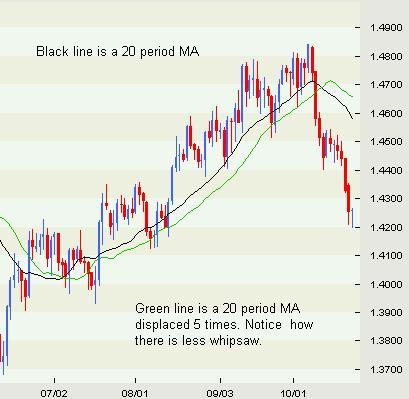There are an infinite number of combinations you could use with displaced moving averages. Experiment for yourself and have some fun with them. When I use moving averages I always use a displacement. I find it just mimics the trend better.

Good Trading

Best Regards
Mark McRae

Information, charts or examples contained in this lesson are for illustration and educational purposes only. It should not be considered as advice or a recommendation to buy or sell any security or financial instrument. We do not and cannot offer investment advice. For further information please read our disclaimer.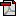To PRINT or save a copy of this lesson in PDF format simply click the PRINT link. This will open the lesson in a PDF format which, you can then PRINT. If you are unfamiliar with PDF or don't have a FREE copy of Arobat Reader see instructions.